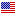1st Edition

# Numerical and Analytical Methods with MATLAB

By William Bober, Chi-Tay Tsai, Oren Masory Copyright 2009
ISBN 9781420093568
488 Pages 267 B/W Illustrations
Published August 11, 2009 by CRC PressFree Shipping (6-12 Business Days)
shipping options

Original Price \$140.00
Sale Price USD \$112.00
ISBN 9780429150937
472 Pages
Published August 11, 2009 by CRC Press

Also available as eBook on:

Numerical and Analytical Methods with MATLAB® presents extensive coverage of the MATLAB programming language for engineers. It demonstrates how the built-in functions of MATLAB can be used to solve systems of linear equations, ODEs, roots of transcendental equations, statistical problems, optimization problems, control systems problems, and stress analysis problems. These built-in functions are essentially black boxes to students. By combining MATLAB with basic numerical and analytical techniques, the mystery of what these black boxes might contain is somewhat alleviated.

This classroom-tested text first reviews the essentials involved in writing computer programs as well as fundamental aspects of MATLAB. It next explains how matrices can solve problems of linear equations, how to obtain the roots of algebraic and transcendental equations, how to evaluate integrals, and how to solve various ODEs. After exploring the features of Simulink, the book discusses curve fitting, optimization problems, and PDE problems, such as the vibrating string, unsteady heat conduction, and sound waves. The focus then shifts to the solution of engineering problems via iteration procedures, differential equations via Laplace transforms, and stress analysis problems via the finite element method. The final chapter examines control systems theory, including the design of single-input single-output (SISO) systems.

Two Courses in One Textbook
The first six chapters are appropriate for a lower level course at the sophomore level. The remaining chapters are ideal for a course at the senior undergraduate or first-year graduate level. Most of the chapters contain projects that require students to write a computer program in MATLAB that produces tables, graphs, or both. Many sample MATLAB programs (scripts) in the text provide guidance on completing these projects.

Numerical Modeling for Engineering

Computer Usage in Engineering

The Mathematical Model

Computer Programming

Preparing a Computer Program

Recommended Procedures for Writing a Program

Building Blocks in Writing a Program

MATLAB Fundamentals

Introduction

Constructing a Program in MATLAB

The MATLAB Program

Program Examples

Debugging a Program

3-D and Contour Plots

Matrices

Matrix Operations

System of Linear Equations

Gauss Elimination

The Gauss–Jordan Method

Number of Solutions

Inverse Matrix

The Eigenvalue Problem

Roots of Algebraic and Transcendental Equations

The Search Method

Bisection Method

Newton–Raphson Method

The fzero Function

Numerical Integration

Numerical Integration and Simpson’s Rule

Improper Integrals

Numerical Integration of Ordinary Differential Equations

The Initial Value Problem

The Fourth-Order Runge–Kutta Method

System of Two First-Order Equations

A Single Second-Order Equation

MATLAB’s ODE Function

Ordinary Differential Equations That Are Not Initial Value Problems

Solution of a Tri-Diagonal System of Linear Equations

Difference Formulas

Deflection of a Beam

Introduction

Typical Building Blocks in Constructing a Model

Constructing and Running the Model

Constructing a Subsystem

Using the mux and fcn Blocks

The Relay Block

The Switch Block

Trigonometric Function Blocks

Curve Fitting

Curve-Fitting Objective

Method of Least Squares

Curve Fitting with the Exponential Function

MATLAB’s Curve-Fitting Function

Cubic Splines

The Function Interp1 for Cubic Spline Curve Fitting

Curve Fitting with Fourier Series

Optimization

Introduction

Unconstrained Optimization Problems

Method of Steepest Descent

Optimization with Constraints

MATLAB’s Optimization Function

Partial Differential Equations

The Classification of Partial Differential Equations

Solution by Separation of Variables

Perturbation Theory and Sound Waves

Review of Finite Difference Formulas

Example of Applying Finite Difference Methods to Partial Differential Equations

Iteration Method

Iteration in Pipe Flow Analysis

The Gauss–Seidel Method

The Hardy–Cross Method

Laplace Transforms

Laplace Transform and Inverse Transform

Transforms of Derivatives

Ordinary Differential Equations, Initial Value Problem

A Shifting Theorem

The Unit Step Function

Laplace Transform of the Unit Step Function

Convolution

Laplace Transforms Applied to Partial Differential Equations

Laplace Transforms and Complex Variables

An Introduction to the Finite Element Method

Finite Element Method for Stress Analysis

Structural Mechanics Plane Stress Analysis

The Shape Function for a Linear Triangle Element

Finite Element Analysis Using MATLAB’s PDE Toolbox

Structural Mechanics Plane Strain Analysis

Model Analysis of 2-D Structures

Finite Element Analysis for Heat Transfer

Control Systems

Introduction

Representation of Systems in MATLAB

Closed-Loop Systems

MATLAB Tools for the Performance Analysis of Closed-Loop Systems

MATLAB’s SISOtool

Application of Simulink in Controls and Dynamic Systems

Appendices

Index

Exercises, Projects, and References appear at the end of most chapters.

### Biography

William Bober, Chi-Tay Tsai, and Oren Masory are all professors in the Department of Mechanical Engineering at Florida Atlantic University.

We offer free standard shipping on every order across the globe.